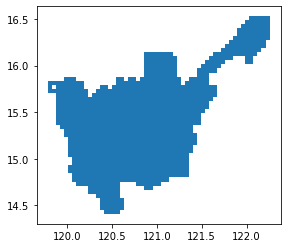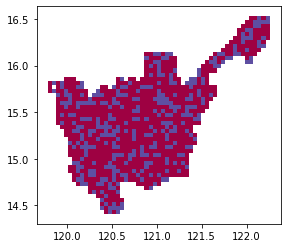## classTileClustering[source]

TileClustering(cluster_type:str='four_way')

Cluster together adjacent square grid cells. Grid cells belonging to the same cluster will get assigned the same ID. Optionally, you cluster adjacent cells by category by passing in category_col

By default, with cluster_type = " four-way", it clusters together grid cells with adjacent edges only. If you wish to consider grid cells with adjacent corners as well, use cluster_type = " eight-way"

#### TileClustering.cluster_tiles[source]

TileClustering.cluster_tiles(df:DataFrame, grid_x_col='x', grid_y_col='y', category_col:Optional[str]=None)

Appends the cluster ID for each square grid cell

## Test data

Create sample scores for square grid cells and cluster the cells

import geopandas as gpd
import numpy as np

from geowrangler import grids

np.random.seed(1562)


grid_generator5k = grids.SquareGridGenerator(5_000)
grid_gdf5k = grid_generator5k.generate_grid(region3_gdf)

x y geometry
0 7 8 POLYGON ((120.10024 14.75528, 120.14516 14.755...
1 6 8 POLYGON ((120.05533 14.75528, 120.10024 14.755...
2 9 8 POLYGON ((120.19008 14.75528, 120.23499 14.755...
3 9 9 POLYGON ((120.19008 14.79871, 120.23499 14.798...
4 10 9 POLYGON ((120.23499 14.79871, 120.27991 14.798...
grid_gdf5k.plot()

<AxesSubplot:>%%time

grid_gdf5k["score"] = np.random.random(len(grid_gdf5k))
grid_gdf5k["class"] = grid_gdf5k["score"] > 0.7

CPU times: user 3.15 ms, sys: 1.32 ms, total: 4.47 ms
Wall time: 10 ms

x y geometry score class
0 7 8 POLYGON ((120.10024 14.75528, 120.14516 14.755... 0.761806 True
1 6 8 POLYGON ((120.05533 14.75528, 120.10024 14.755... 0.012455 False
2 9 8 POLYGON ((120.19008 14.75528, 120.23499 14.755... 0.446552 False
3 9 9 POLYGON ((120.19008 14.79871, 120.23499 14.798... 0.669020 False
4 10 9 POLYGON ((120.23499 14.79871, 120.27991 14.798... 0.815914 True
%%time
tileclustering = TileClustering()
grid_gdf5k = tileclustering.cluster_tiles(grid_gdf5k, category_col="class")

CPU times: user 25.8 ms, sys: 0 ns, total: 25.8 ms
Wall time: 47.6 ms

x y geometry score class tile_cluster
0 7 8 POLYGON ((120.10024 14.75528, 120.14516 14.755... 0.761806 True 6-1
1 6 8 POLYGON ((120.05533 14.75528, 120.10024 14.755... 0.012455 False 7-2
2 9 8 POLYGON ((120.19008 14.75528, 120.23499 14.755... 0.446552 False 1-2
3 9 9 POLYGON ((120.19008 14.79871, 120.23499 14.798... 0.669020 False 1-2
4 10 9 POLYGON ((120.23499 14.79871, 120.27991 14.798... 0.815914 True 23-1
grid_gdf5k["tile_cluster"].nunique()

160
grid_gdf5k.plot(column="class", categorical=True, cmap="Spectral")

<AxesSubplot:>